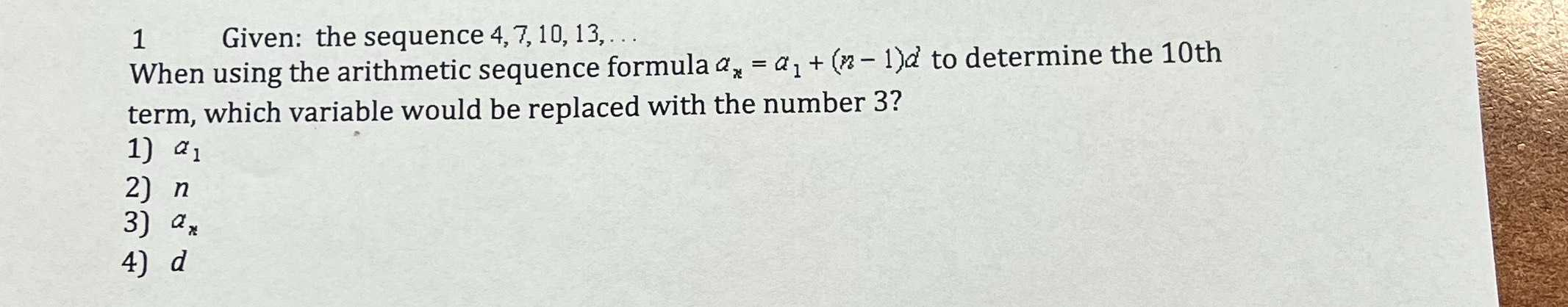### ¿Todavía tienes preguntas de matemáticas?

Pregunte a nuestros tutores expertos
Algebra
Pregunta$$1$$ Given: the sequence $$4,7,10,13 , \ldots$$

When using the arithmetic sequence formula $$a _ { n } = a _ { 1 } + ( n - 1 ) d$$ to determine the $$10$$ th term, which variable would be replaced with the number $$3$$ ?

1) $$a _ { 1 }$$

2) $$n$$

3) $$a _ { n }$$

4) $$d$$